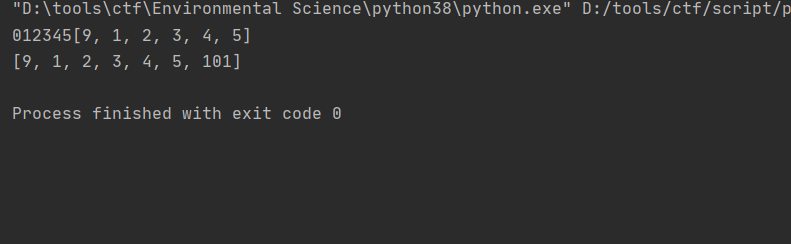# 数据结构实现数组

``````* 抽象数据类型 ADT，面向对象编程
* 数组和列表
* 链表，高级链表。双链表，循环双端链表
* 队列，双端队列，循环双端队列
* 栈，栈溢出
* 算法分析，时间复杂度 大O 表示法
* 哈希表，散列冲突
* 字典
* 集合
* 递归
* 查找：线性查找和二分查找
* 基本排序算法
* 高级排序算法: 归并排序、堆排序、快排
* 树，二叉树
* 图，dfs 和 bfs
* python 内置常用数据结构和算法的使用。list, dict, set, collections 模块，heapq 模块
* 面试笔试常考算法
``````

## 使用python实现数组

``````class Array(object):
def __init__(self,capacity,fillvalue=None):
self.items=list()
for count in range(capacity):
self.items.append(fillvalue)

def editarray(self,index):
if index+1>int(len(self.items)):
#当当前索引下位标识超过数组长度自动添加数组长度
temp=Array(index+1)
for p in range(0,len(self.items)):
temp.__setitem__(p,self.items[p])
self.items=temp
del temp
return True
else:
return False

def __len__(self):
#返回数组长度
return len(self.items)
def __str__(self):
#返回str类型的数组
return str(self.items)
def __iter__(self):
#返回遍历好的数组
return iter(self.items)
def __getitem__(self, index):
#通过索引获取数组中的值
return self.items[index]
def __setitem__(self, index, newItem):
#替换指定位置的值
self.editarray(index)
self.items[index]=newItem
return self.items

def __delitem__(self, key):
#删除数组指定下标
del self.items[key]
return self.items
#数据末尾添加
calc=0
for k in self.items:
if len(str(k))!=0:
calc+=1
else:
break

self.__setitem__(calc,value)

if __name__ == '__main__':
a=Array(6)
for i in range(7):
a[i]=i
a.__delitem__(-1)
for x in a.__iter__():
print(x,end='')
a.__setitem__(0,9)
print(a.__str__())
print(a.__str__())
``````